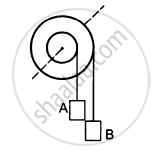Department of Pre-University Education, KarnatakaPUC Karnataka Science Class 11
Advertisement Remove all ads

# The Following Figure Shows a Small Wheel Fixed Coaxially on a Bigger One of Double the Radius. the System Rotates About the Common Axis - Physics

MCQ
Fill in the Blanks

The following figure shows a small wheel fixed coaxially on a bigger one of double the radius. The system rotates about the common axis. The strings supporting A and B do not slip on the wheels. If x and y be the distance travelled by A and B in the same time interval, then _________ .#### Options

• x = 2y

• x = y

• y = 2x

• None of these

Advertisement Remove all ads

#### Solution

y = 2x

It is given that angular velocity is same for both the wheels.

Therefore, we have

vA = $\omega$ R

vB =$\omega$2R

x = vAt = $\omega$Rt ............(1)

y = vBt = $\omega$(2R)t ..............(2)

From equations (1) and (2), we get

y = 2x

Is there an error in this question or solution?
Advertisement Remove all ads

#### APPEARS IN

HC Verma Class 11, 12 Concepts of Physics 1
Chapter 10 Rotational Mechanics
MCQ | Q 5 | Page 193
Advertisement Remove all ads
Advertisement Remove all ads
Share
Notifications

View all notifications

Forgot password?